# 4 APPLICATIONS OF DIFFERENTIATION APPLICATIONS OF DIFFERENTIATION We

• Slides: 394 APPLICATIONS OF DIFFERENTIATIONAPPLICATIONS OF DIFFERENTIATION We will see that many of the results of this chapter depend on one central fact—the Mean Value Theorem.APPLICATIONS OF DIFFERENTIATION 4. 2 The Mean Value Theorem In this section, we will learn about: The significance of the mean value theorem.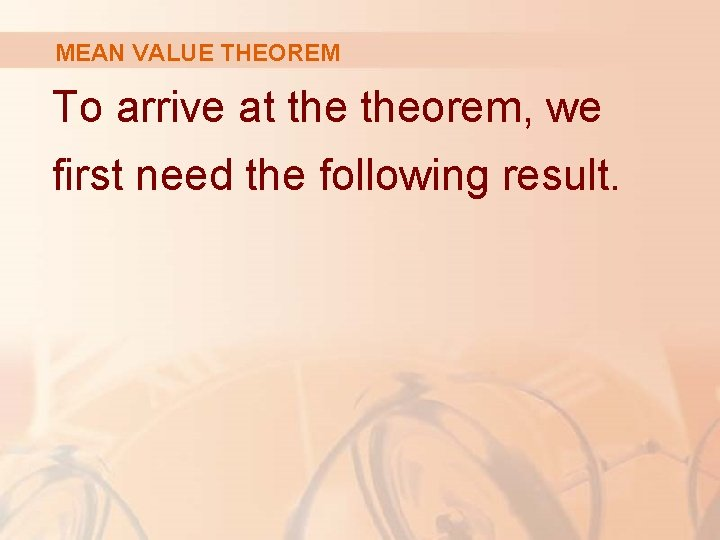MEAN VALUE THEOREM To arrive at theorem, we first need the following result.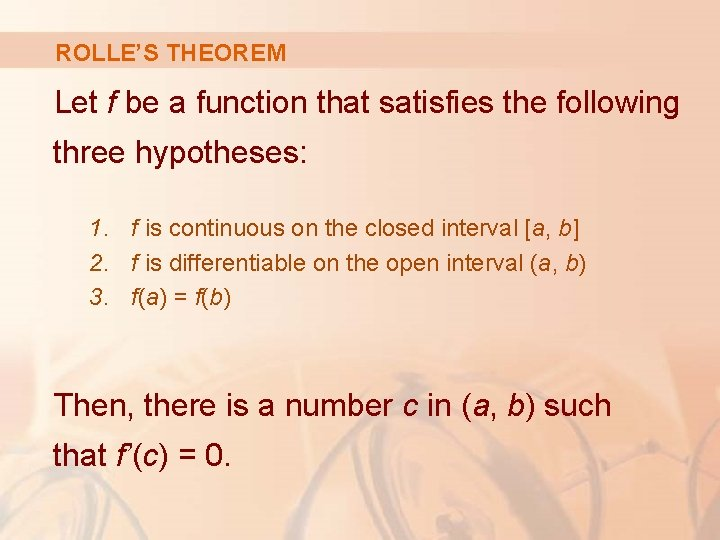ROLLE’S THEOREM Let f be a function that satisfies the following three hypotheses: 1. f is continuous on the closed interval [a, b] 2. f is differentiable on the open interval (a, b) 3. f(a) = f(b) Then, there is a number c in (a, b) such that f’(c) = 0.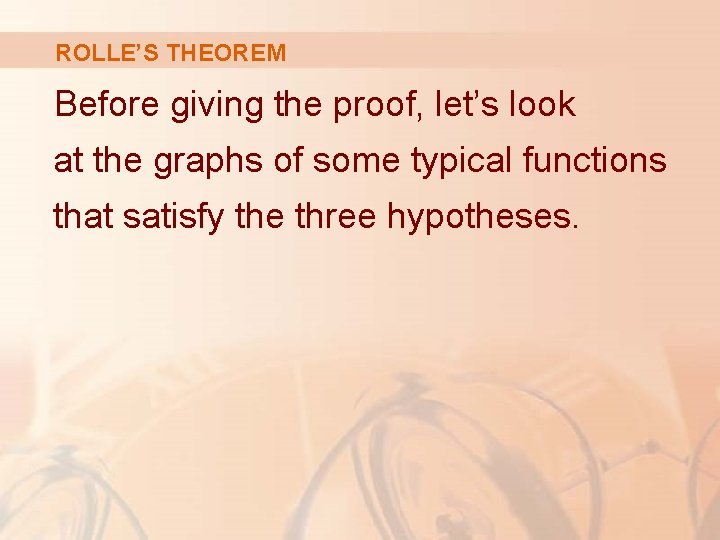ROLLE’S THEOREM Before giving the proof, let’s look at the graphs of some typical functions that satisfy the three hypotheses.ROLLE’S THEOREM The figures show the graphs of four such functions.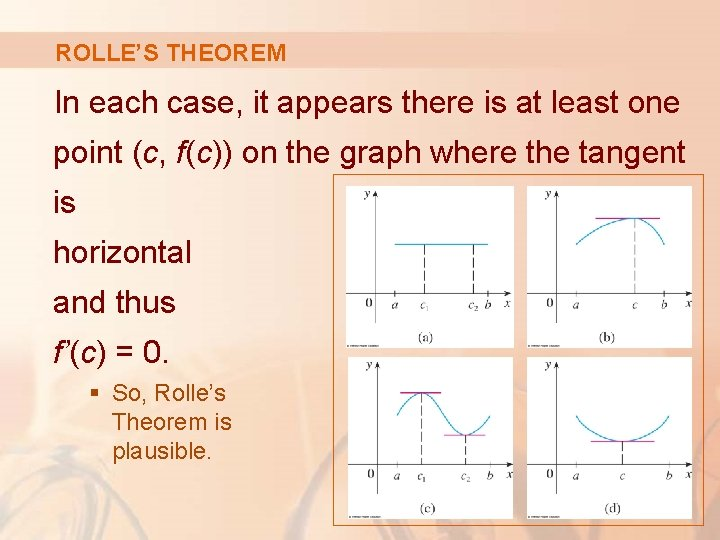ROLLE’S THEOREM In each case, it appears there is at least one point (c, f(c)) on the graph where the tangent is horizontal and thus f’(c) = 0. § So, Rolle’s Theorem is plausible.ROLLE’S THEOREM Proof There are three cases: 1. f(x) = k, a constant 2. f(x) > f(a) for some x in (a, b) 3. f(x) < f(a) for some x in (a, b)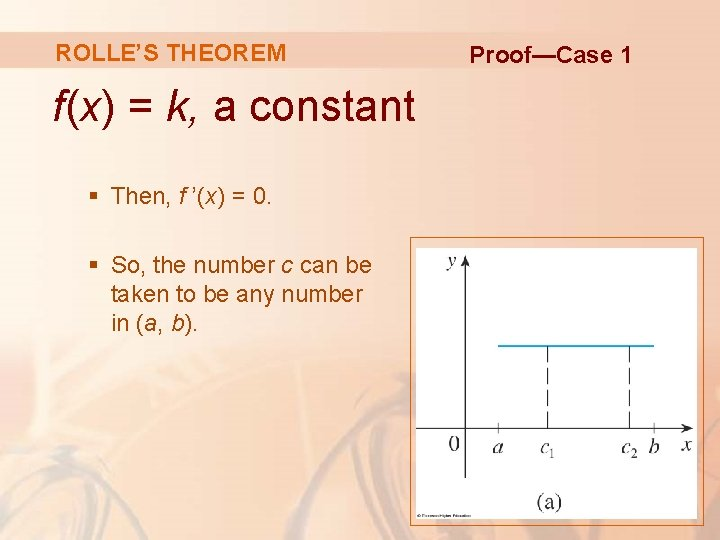ROLLE’S THEOREM f(x) = k, a constant § Then, f ’(x) = 0. § So, the number c can be taken to be any number in (a, b). Proof—Case 1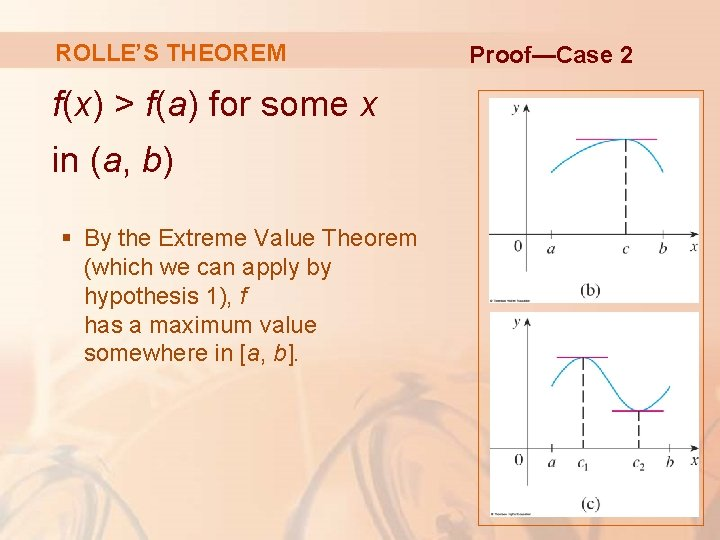ROLLE’S THEOREM f(x) > f(a) for some x in (a, b) § By the Extreme Value Theorem (which we can apply by hypothesis 1), f has a maximum value somewhere in [a, b]. Proof—Case 2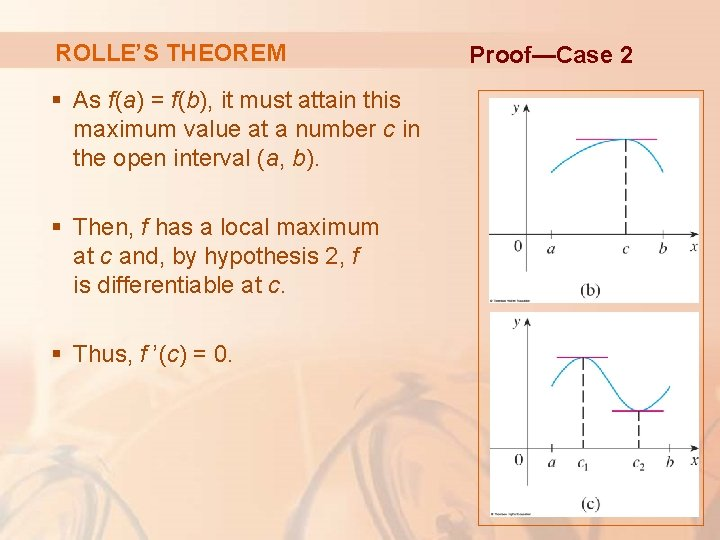ROLLE’S THEOREM § As f(a) = f(b), it must attain this maximum value at a number c in the open interval (a, b). § Then, f has a local maximum at c and, by hypothesis 2, f is differentiable at c. § Thus, f ’(c) = 0. Proof—Case 2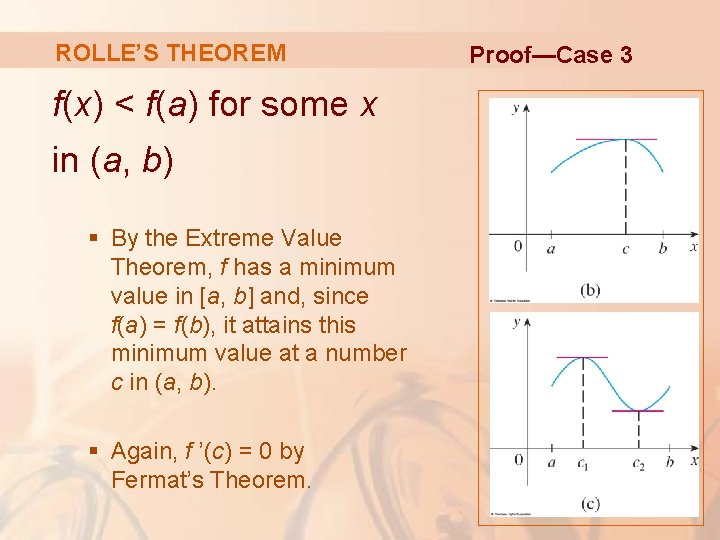ROLLE’S THEOREM f(x) < f(a) for some x in (a, b) § By the Extreme Value Theorem, f has a minimum value in [a, b] and, since f(a) = f(b), it attains this minimum value at a number c in (a, b). § Again, f ’(c) = 0 by Fermat’s Theorem. Proof—Case 3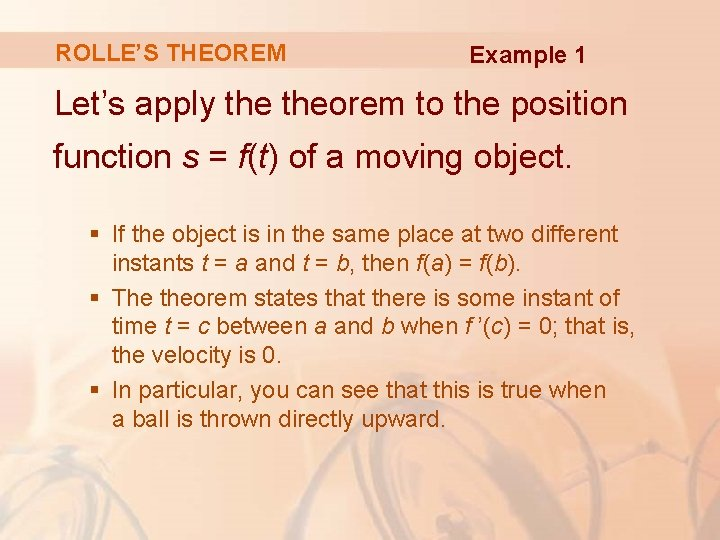ROLLE’S THEOREM Example 1 Let’s apply theorem to the position function s = f(t) of a moving object. § If the object is in the same place at two different instants t = a and t = b, then f(a) = f(b). § The theorem states that there is some instant of time t = c between a and b when f ’(c) = 0; that is, the velocity is 0. § In particular, you can see that this is true when a ball is thrown directly upward.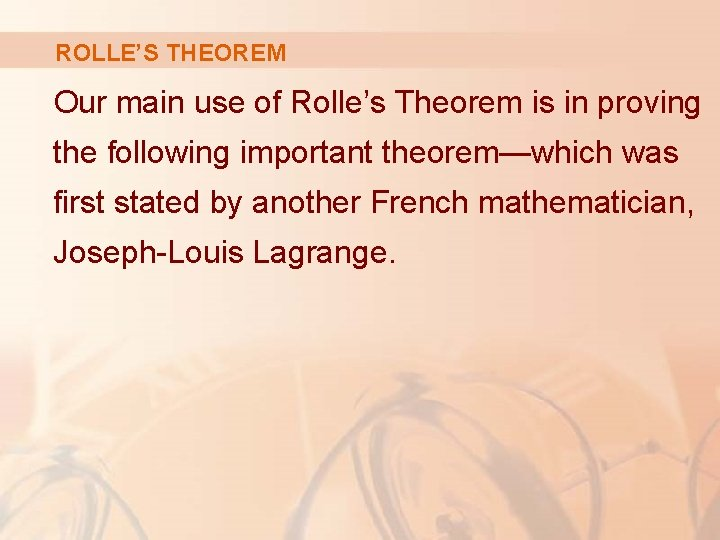ROLLE’S THEOREM Our main use of Rolle’s Theorem is in proving the following important theorem—which was first stated by another French mathematician, Joseph-Louis Lagrange.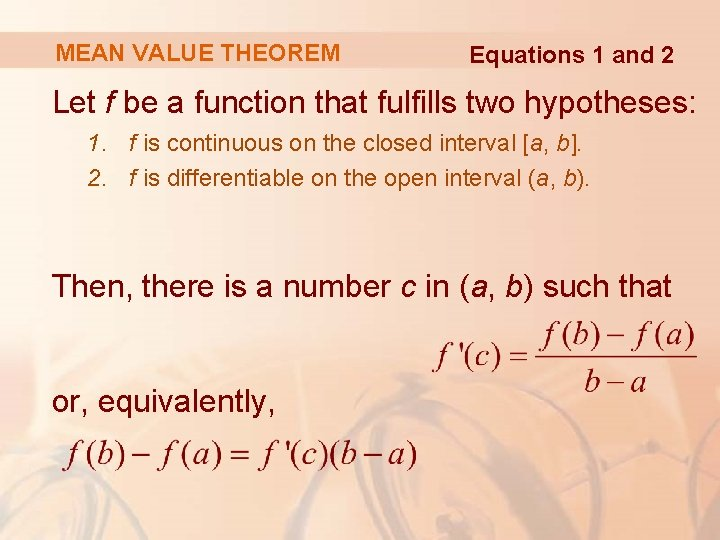MEAN VALUE THEOREM Equations 1 and 2 Let f be a function that fulfills two hypotheses: 1. f is continuous on the closed interval [a, b]. 2. f is differentiable on the open interval (a, b). Then, there is a number c in (a, b) such that or, equivalently,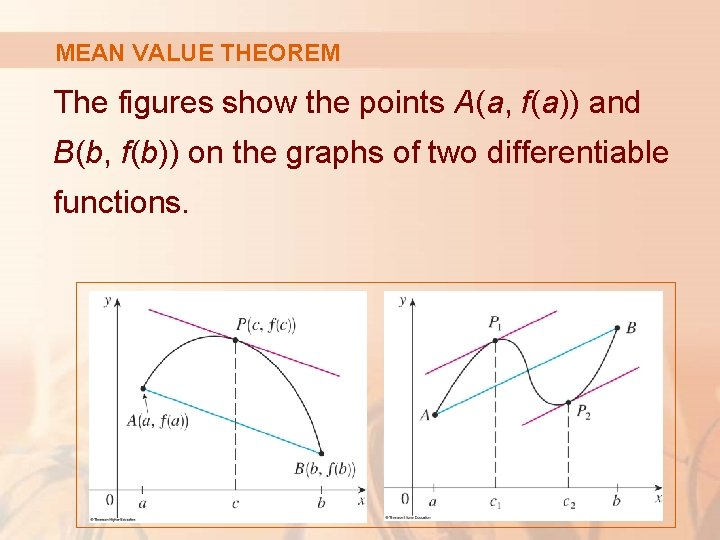MEAN VALUE THEOREM The figures show the points A(a, f(a)) and B(b, f(b)) on the graphs of two differentiable functions.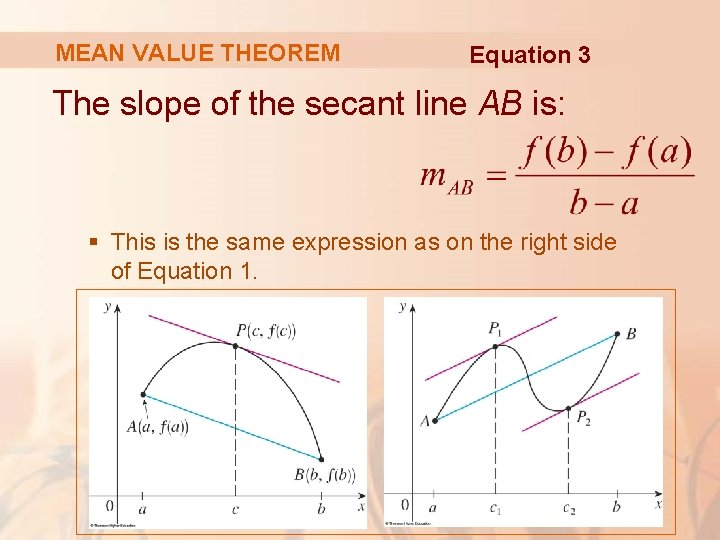MEAN VALUE THEOREM Equation 3 The slope of the secant line AB is: § This is the same expression as on the right side of Equation 1.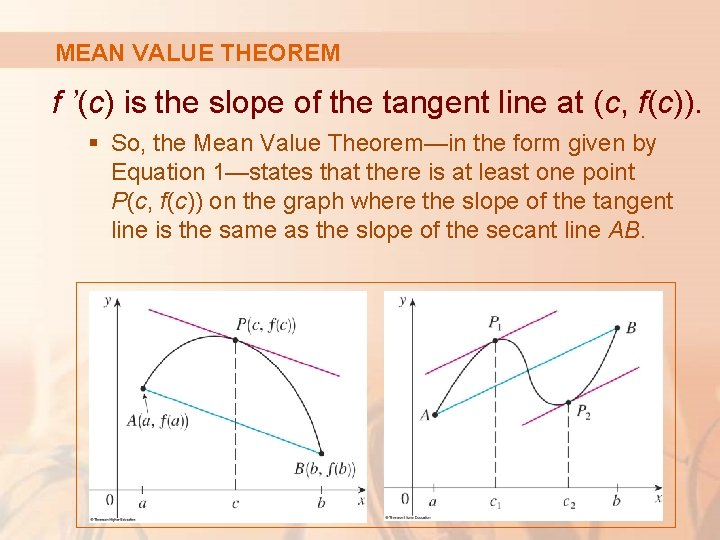MEAN VALUE THEOREM f ’(c) is the slope of the tangent line at (c, f(c)). § So, the Mean Value Theorem—in the form given by Equation 1—states that there is at least one point P(c, f(c)) on the graph where the slope of the tangent line is the same as the slope of the secant line AB.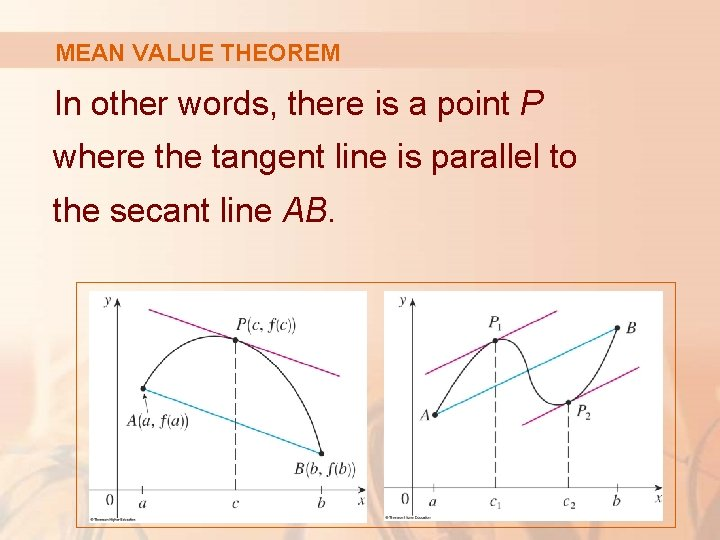MEAN VALUE THEOREM In other words, there is a point P where the tangent line is parallel to the secant line AB.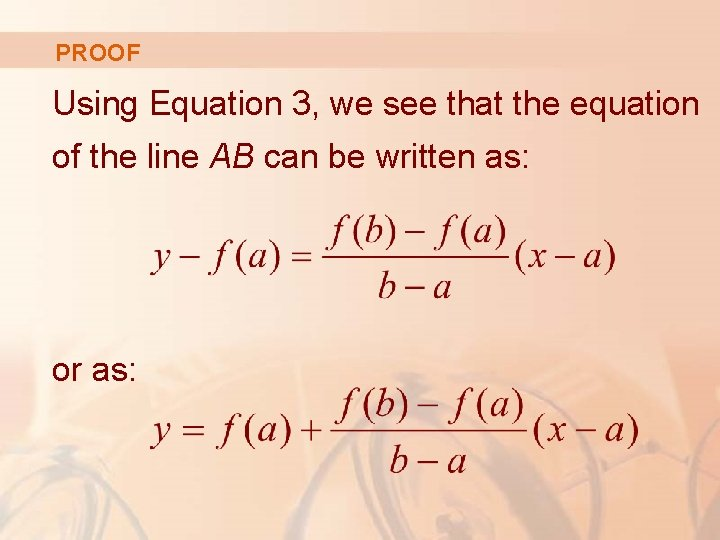PROOF Using Equation 3, we see that the equation of the line AB can be written as: or as: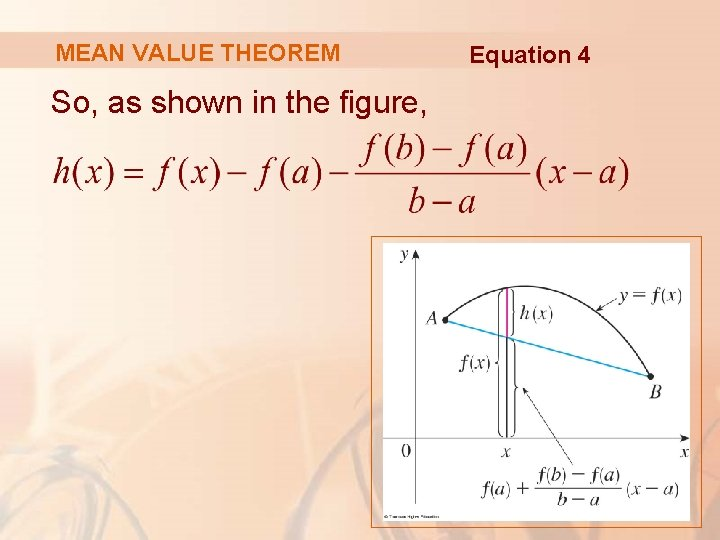MEAN VALUE THEOREM So, as shown in the figure, Equation 4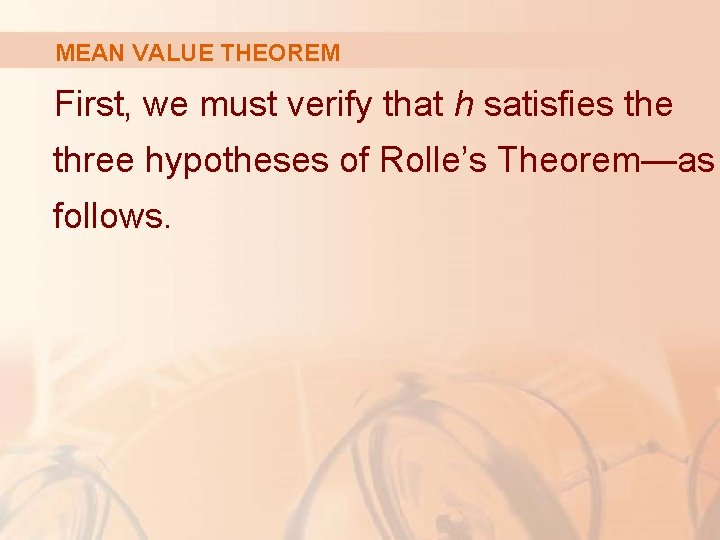MEAN VALUE THEOREM First, we must verify that h satisfies the three hypotheses of Rolle’s Theorem—as follows.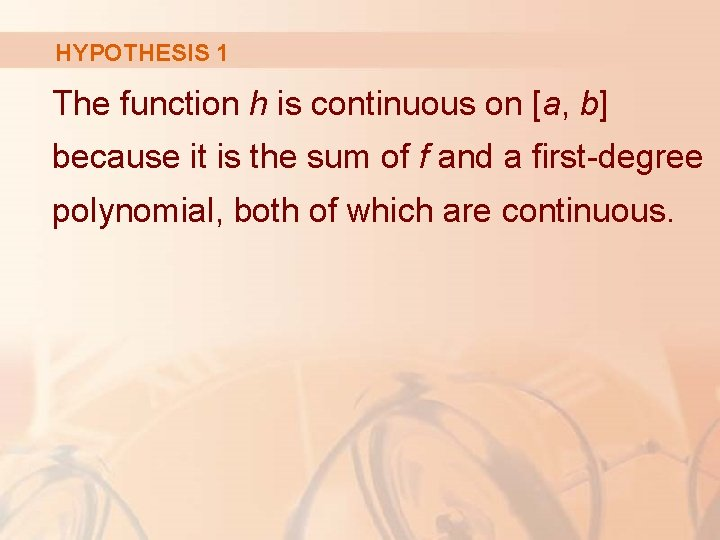HYPOTHESIS 1 The function h is continuous on [a, b] because it is the sum of f and a first-degree polynomial, both of which are continuous.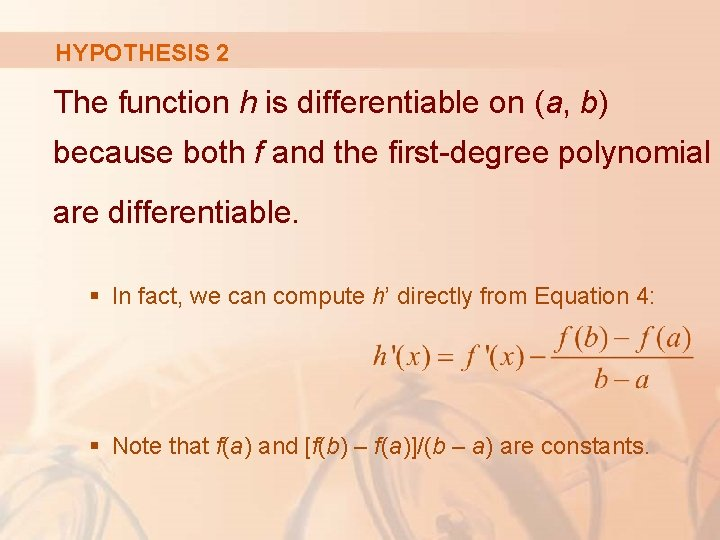HYPOTHESIS 2 The function h is differentiable on (a, b) because both f and the first-degree polynomial are differentiable. § In fact, we can compute h’ directly from Equation 4: § Note that f(a) and [f(b) – f(a)]/(b – a) are constants.HYPOTHESIS 3 Therefore, h(a) = h(b).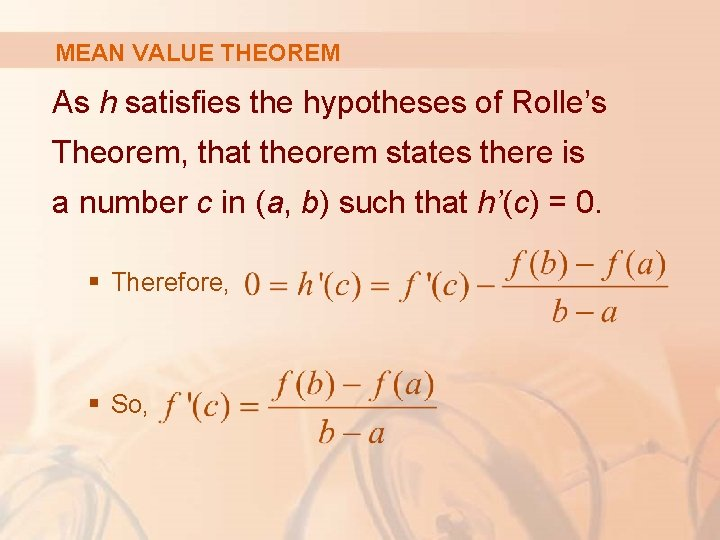MEAN VALUE THEOREM As h satisfies the hypotheses of Rolle’s Theorem, that theorem states there is a number c in (a, b) such that h’(c) = 0. § Therefore, § So,MEAN VALUE THEOREM Example 3 To illustrate the Mean Value Theorem with a specific function, let’s consider f(x) = x 3 – x, a = 0, b = 2.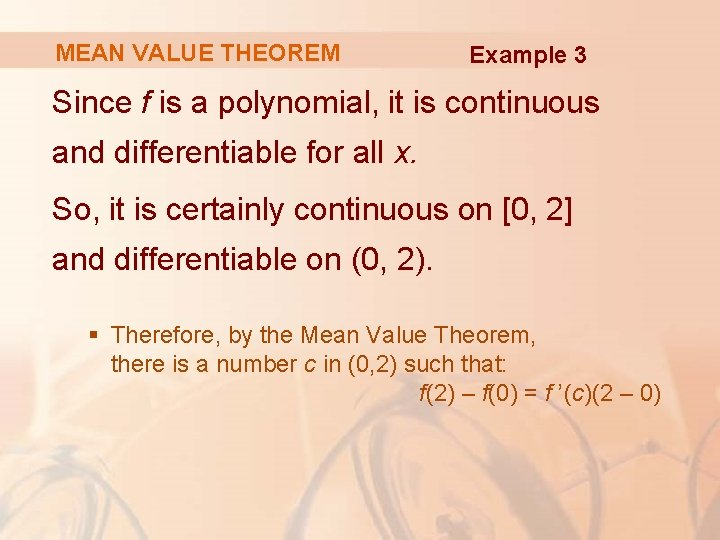MEAN VALUE THEOREM Example 3 Since f is a polynomial, it is continuous and differentiable for all x. So, it is certainly continuous on [0, 2] and differentiable on (0, 2). § Therefore, by the Mean Value Theorem, there is a number c in (0, 2) such that: f(2) – f(0) = f ’(c)(2 – 0)MEAN VALUE THEOREM Example 3 Now, f(2) = 6, f(0) = 0, and f ’(x) = 3 x 2 – 1. So, this equation becomes 6 = (3 c 2 – 1)2 = 6 c 2 – 2 § This gives c 2 = , that is, c = § However, c must lie in (0, 2), so c = . .MEAN VALUE THEOREM Example 3 The figure illustrates this calculation. § The tangent line at this value of c is parallel to the secant line OB.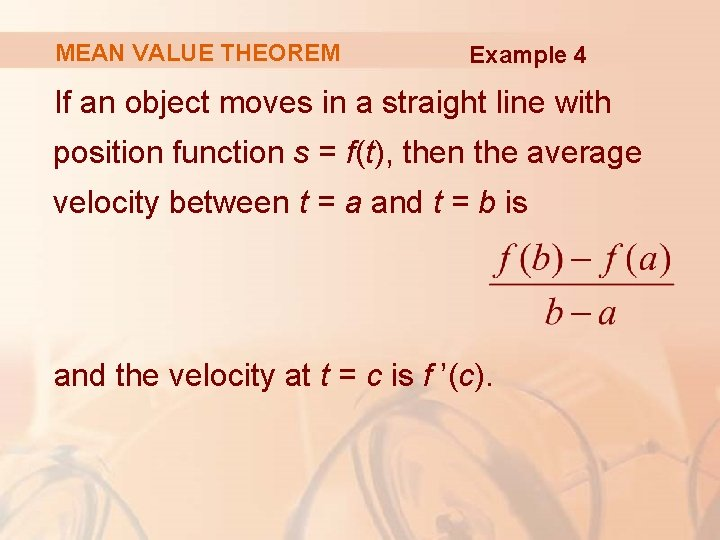MEAN VALUE THEOREM Example 4 If an object moves in a straight line with position function s = f(t), then the average velocity between t = a and t = b is and the velocity at t = c is f ’(c).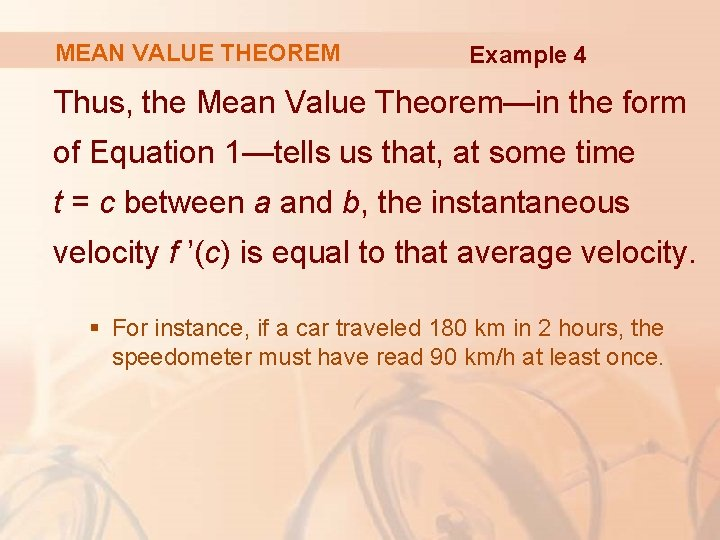MEAN VALUE THEOREM Example 4 Thus, the Mean Value Theorem—in the form of Equation 1—tells us that, at some time t = c between a and b, the instantaneous velocity f ’(c) is equal to that average velocity. § For instance, if a car traveled 180 km in 2 hours, the speedometer must have read 90 km/h at least once.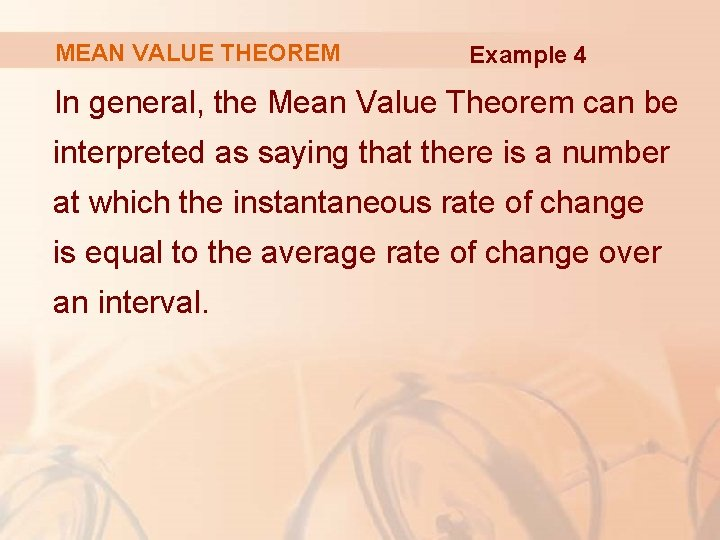MEAN VALUE THEOREM Example 4 In general, the Mean Value Theorem can be interpreted as saying that there is a number at which the instantaneous rate of change is equal to the average rate of change over an interval.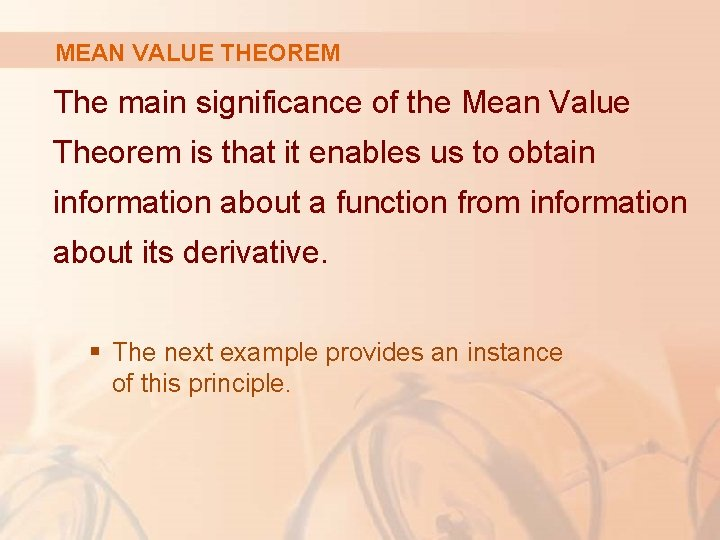MEAN VALUE THEOREM The main significance of the Mean Value Theorem is that it enables us to obtain information about a function from information about its derivative. § The next example provides an instance of this principle.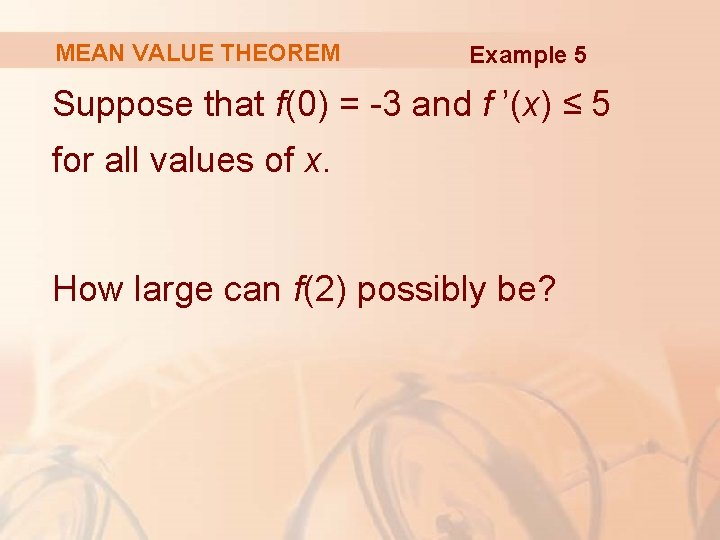MEAN VALUE THEOREM Example 5 Suppose that f(0) = -3 and f ’(x) ≤ 5 for all values of x. How large can f(2) possibly be?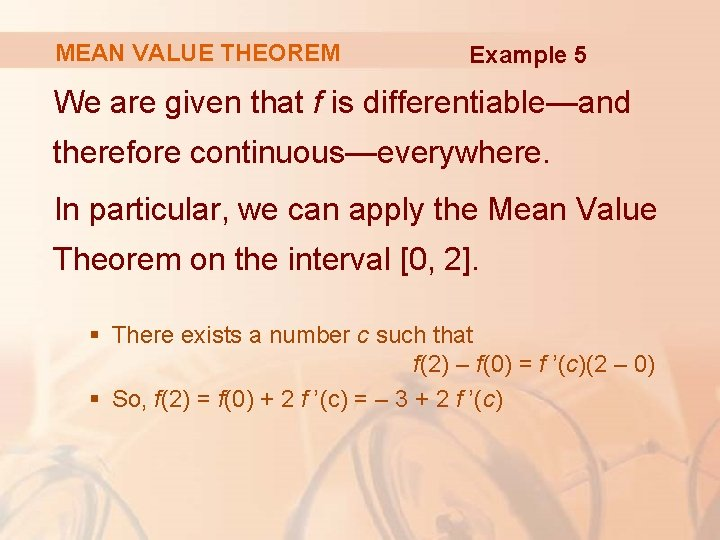MEAN VALUE THEOREM Example 5 We are given that f is differentiable—and therefore continuous—everywhere. In particular, we can apply the Mean Value Theorem on the interval [0, 2]. § There exists a number c such that f(2) – f(0) = f ’(c)(2 – 0) § So, f(2) = f(0) + 2 f ’(c) = – 3 + 2 f ’(c)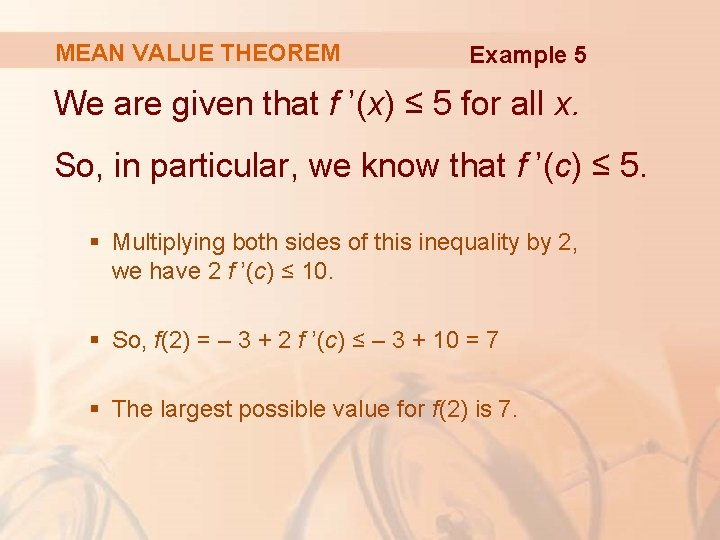MEAN VALUE THEOREM Example 5 We are given that f ’(x) ≤ 5 for all x. So, in particular, we know that f ’(c) ≤ 5. § Multiplying both sides of this inequality by 2, we have 2 f ’(c) ≤ 10. § So, f(2) = – 3 + 2 f ’(c) ≤ – 3 + 10 = 7 § The largest possible value for f(2) is 7.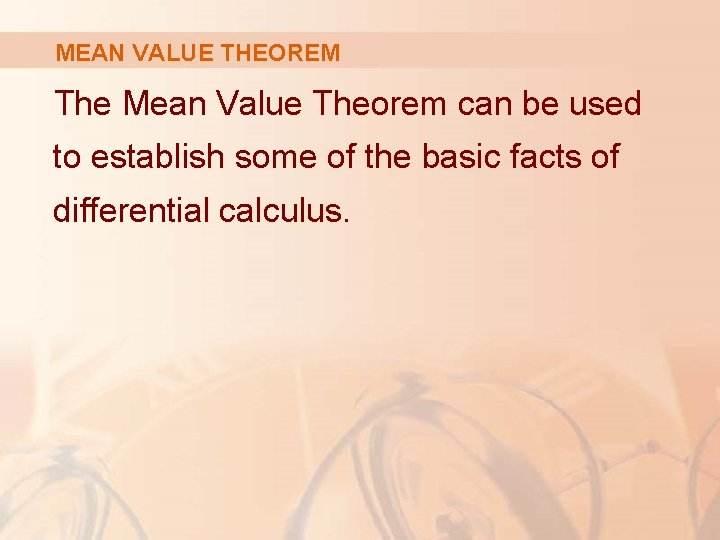MEAN VALUE THEOREM The Mean Value Theorem can be used to establish some of the basic facts of differential calculus.